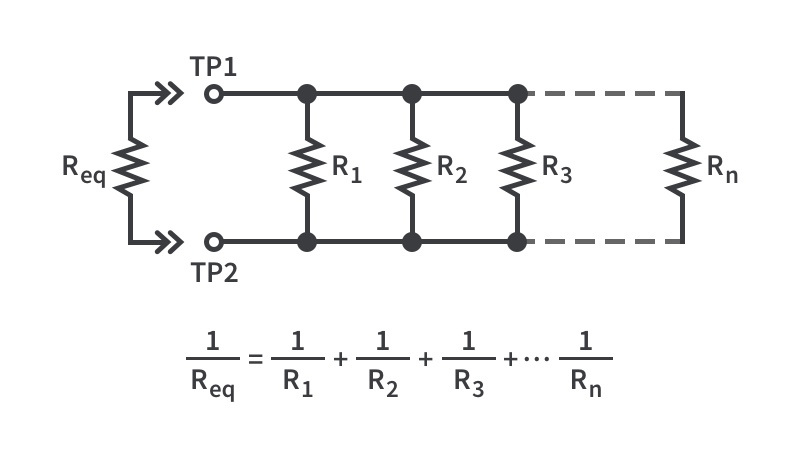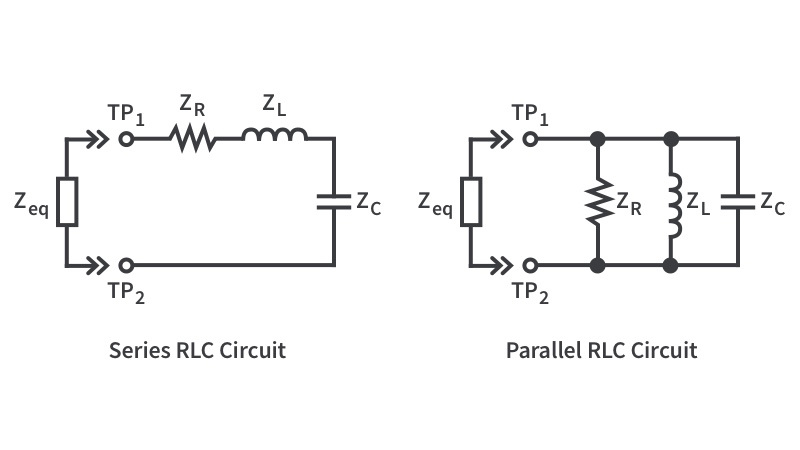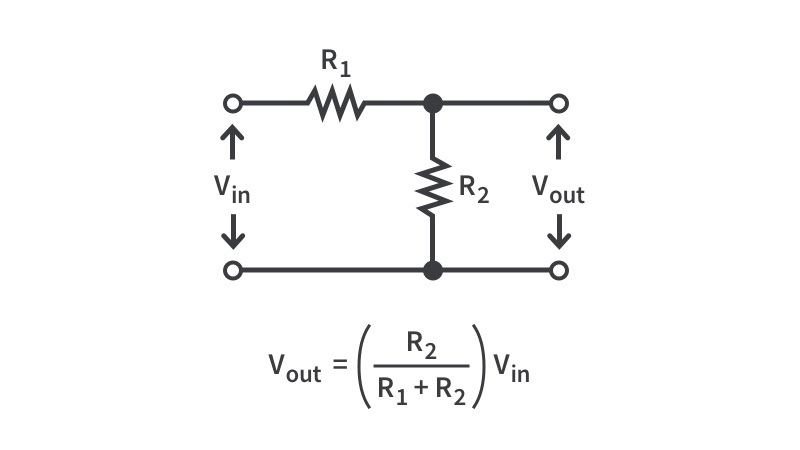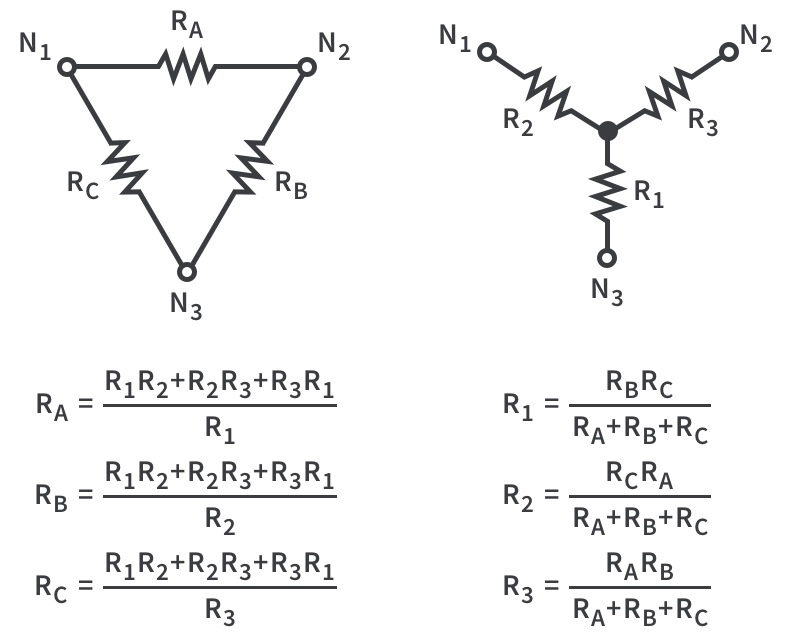Electronics Tools

Mobile friendly electronics tools and reference materials.## Electronics ToolBox

40 Tools

Welcome to our electronics toolbox! In this toolbox, we have many tools to help hobbyist and professionals with their electronics calculations. Solve typical circuit configurations with our parallel and series equivalence calculators, voltage divider calculator, and delta-wye calculator or find the Q points and resonant frequencies with our RLC calculator, and finally, take advantage of our free, powerful, and easy-to-use scientific calculator.

In addition to these tools, we have unit converters for a wide variety of electronics and non-electronics units, from energy and power unit conversion to volume and speed unit converters. Are there a few converters that you would like to use frequently? Login and highlight them to save them to your profile for easy access.

Finally, we’re continuously adding new, ready-to-print reference materials as we work through different topics while creating tutorials. These references let you get quick access to important information in those topics in a clean and clear way.

We’re constantly trying to come up with the best calculators to help people of all skill levels and make them both desktop and mobile friendly. If anything is broken or you have any ideas for tools that we need to make our collection complete, reach out to us and let us know!

## Featured Electronics CalculatorsParallel Equivalence

Calculating the parallel equivalence of a resistor, especially when there are more than two of them, is certainly more complicated than finding the series equivalence. However, you can use the formula above to do it yourself and then check to make sure you did it correctly with this convenient calculator!RLC Calculator

When you have a resistor, inductor, and capacitor in the same circuit, the way that circuit reacts at different frequencies can change dramatically. At low frequencies, the capacitor acts as an open and the inductor acts as a short. At high frequency, this flips with the capacitor acting as a short and the inductor as an open. That’s easy. But it gets weird when trying to figure out at what point one dominates the other. Using this calculator, you can find the resonant frequency, which means that you can disregard the reactive impedance (reactance) and only pay attention to the resistive impedance (resistance). What this means to you is that at the resonant frequency, depending on whether the circuit is in parallel or series, the impedance is the highest or the lowest, respectively.

In summary:

Resonant frequency for parallel circuit - frequency at which the impedance of the circuit is highest.

Resonant frequency for series circuit - frequency at which the impedance of the circuit is lowest.Voltage Divider

A simple, dirty way to get a lower voltage is to use a voltage divider - putting two (or more!) resistors in series and tapping the voltage between them. If you know the value of the resistors and voltage input, you can calculate the output voltage. If the resistors are the same value, then you’ll get half the input voltage. Otherwise, the math is going to be slightly more complicated but you won’t have any issues - just use the equation above and then use this fancy tool to double-check your work! Or just use the tool, we’re cool with that, too.Delta-Wye Calculator

Wye, oh wye, would we need a calculator like this? Well, if you do much with three phase power, it could be very helpful to transform a wye circuit into a delta (or pi) circuit or vice versa. As you can see from the equations above, these calculations aren’t necessarily hard but they are tedious. By using this calculator, it should make things much faster for you!Get the latest tools and tutorials, fresh from the toaster.

### What are you looking for?